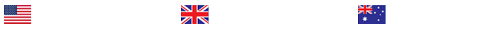Create and compute summary statistics for a dataset provided as a Microsoft Excel file. Import the data into SPSS and then calculate the summary statistics, including the mean, median, mode, range, range, and the standard deviation

Application: Analyzing Summary Statistics
Your goal for this Application is to compute the summary statistics for a data set provided. Use SPSS to calculate the summary statistics, including the mean, median, mode, range, quartiles, interquartile range, semi-interquartile range, and the standard deviation.
A worksheet is provided for this Application in the Learning Resources, in the form of a Microsoft Word document. Download the Module Application Assignment Worksheet and paste your output—the summary statistics you calculated—at the end of the document. Then, based on your output, answer the questions on the worksheet, typing your answers directly into the document.
To prepare for this Application, participate in the Discussion area to clarify any questions you may have about the material presented in the textbook, demonstration video, the assigned Study Questions, or the end-of-chapter exercises.
The Assignment:
Complete the Module Application Assignment Worksheet, pasting your summary statistics output at the end of the document. Be sure that your completed worksheet contains your responses to all questions and your SPSS output.
Module Application Assignment Worksheet
SPSS Summary Statistics

Instructions
For this assignment, you create and compute summary statistics for a dataset provided as a Microsoft Excel file. Import the data into SPSS and then calculate the summary statistics, including the mean, median, mode, range, range, and the standard deviation as instructed below. Note: If you correctly saved the data file from Module 4 assignment, you may open and use that saved file to complete this assignment. Type your answers to all questions directly into the worksheet, and paste the required summary statistics output at the end of this document.
Research Scenario

A researcher is interested in the effect of a new medication on serum cholesterol, HDL cholesterol, and glycosylated hemoglobin of adults. The researcher randomly selects a sample of 40 (20 male and 20 female) participants who have been diagnosed with high cholesterol. Assuring equal distribution of males and females, the participants are randomly assigned to one of two conditions (or groups): Following pretest measures of serum cholesterol (CHOL), High density lipoprotein cholesterol (HDL), and glycosylated hemoglobin (GLYHB), the experimental group (group 1) is given the medication for a period of six months while the control group (group 2) is given a placebo. After the six months, CHOL, HDL and GLYHB are again measured.

The post-test data for each participant are provided in the data set “Module 4, 5, and 6 applic assign data_Cholesterol etc” and can be found in the module learning resources. The codebook for the data provided is as follows:

AGE Age in years
SEX 1 =male, 2=female
GROUP 1 =medication, 2=placebo
CHNG_CHOL change in cholesterol from pre-test to post-test
HDL High density lipoprotein at post-test
GLYHB Glycosylated hemoglobin at post-test
———————————————————————————————————————

Step 1: Import the Microsoft Excel data file into SPSS or use the correct saved SPSS data file as noted in the instructions, above.

Step 2: Run descriptive/summary statistics (mean, median, mode, standard deviation, and range) for both groups 1 and 2 (medication and placebo), combined for each of the following: AGE, CHNG_CHOL,HDL, and GLYHB (be sure to save your output)

Step 3: Separate the data file by group
Step 4: Run descriptive/summary statistics for each group separately for each of the following: AGE, CHNG_CHOL,HDL, and GLYHB (be sure to save your output)

Step 5: Review your SPSS output and answer each of the following questions:

For groups 1 and 2 (medication and placebo) combined:

1. What is the mean for AGE? ________________
2. What is the median for AGE? ________________
3. What is the standard deviation for AGE? ________________
4. What is the mean for CHANGE_CHOL? ________________
5. What is the median for CHANGE_CHOL? ________________
6. What is the standard deviation for CHANGE_CHOL? ________________
7. What is the mean for HDL? ________________
8. What is the median for HDL? ________________
9. What is the standard deviation for HDL? ________________
10. What is the mean for GLYHB? ________________
11. What is the median for GLYHB? ________________
12. What is the standard deviation GLYHB? ________________
For group 1 (medication) only:

13. What is the mean for CHANGE_CHOL? ________________
14. What is the median for CHANGE_CHOL? ________________
15. What is the standard deviation for CHANGE_CHOL? ________________
16. What is the range for CHANGE_CHOL? ________________
For group 2 (placebo) only:

17. What is the mean for CHANGE_CHOL? ________________
18. What is the median for CHANGE_CHOL? ________________

19.What is the standard deviation for CHANGE_CHOL? ________________
20.0What is the range for CHANGE_CHOL? ________________
Step 6: Paste all required SPSS output below.

### Last Completed Projects

# topic title discipline academic level pages delivered
6
Writer's choice
University
2
1 hour 32 min
7
Wise Approach to
Philosophy
College
2
2 hours 19 min
8
1980's and 1990
History
College
3
2 hours 20 min
9
pick the best topic
Finance
School
2
2 hours 27 min
10
finance for leisure
Finance
University
12
2 hours 36 min